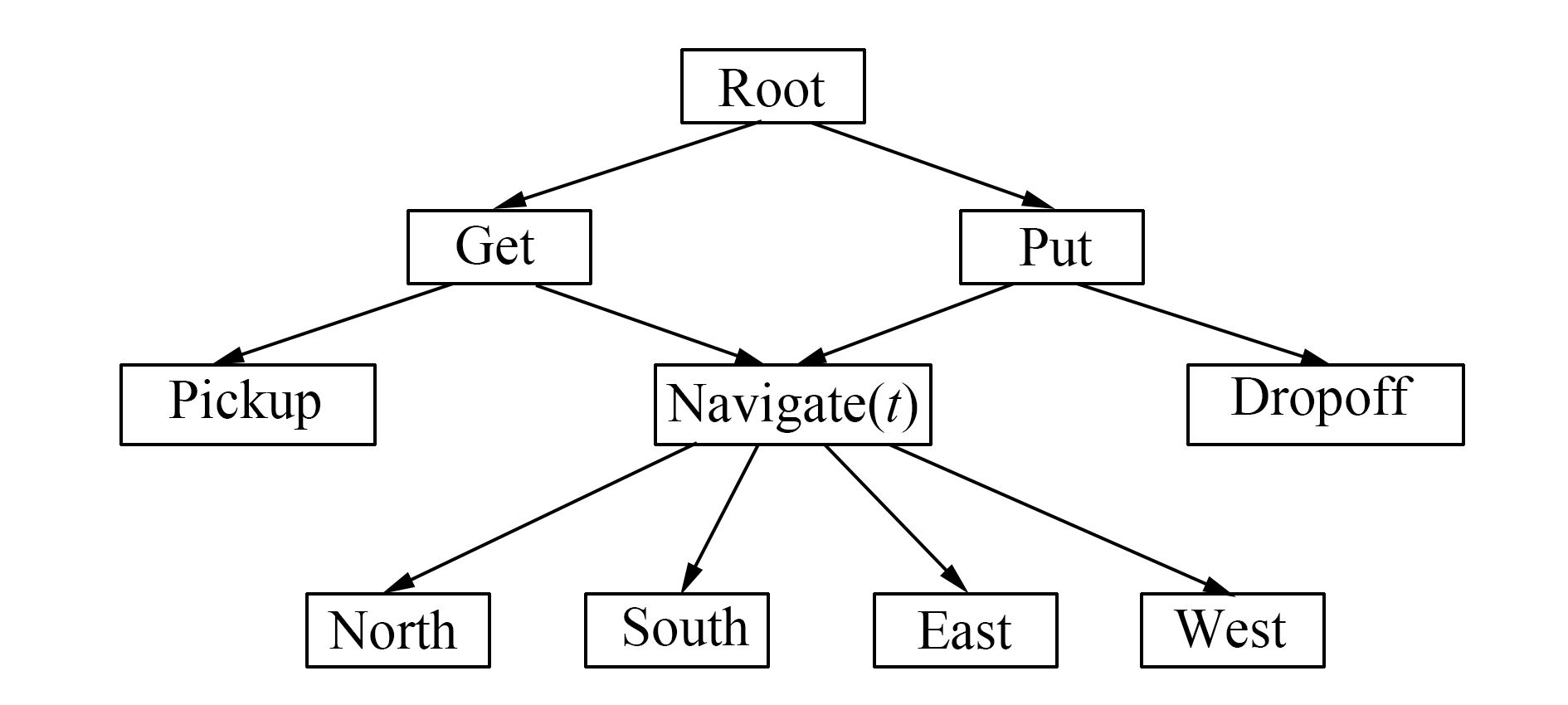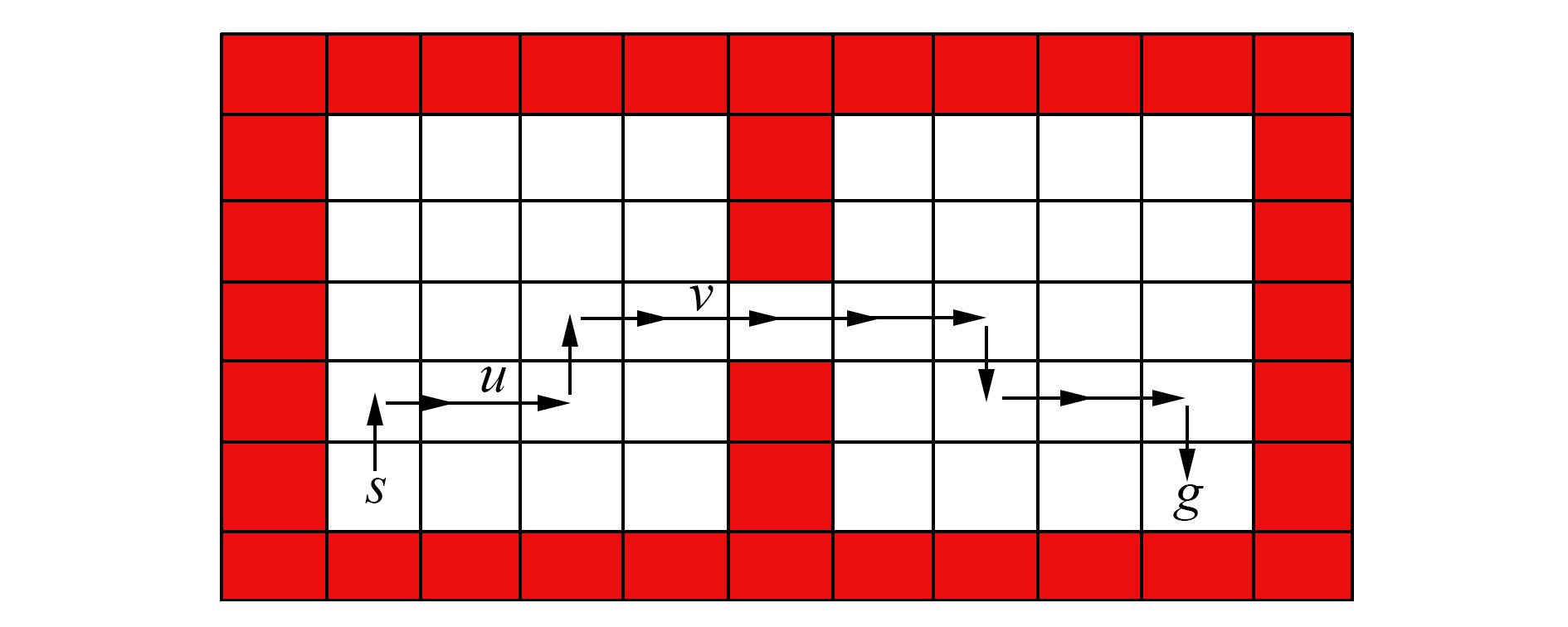﻿ 分层强化学习综述
«上一篇文章快速检索 高级检索

 智能系统学报2017, Vol. 12Issue (5): 590-594  DOI: 10.11992/tis.2017060310

### 引用本文ZHOU Wenji, YU Yang. Summarize of hierarchical reinforcement learning[J]. CAAI Transactions on Intelligent Systems, 2017, 12(5): 590-594. DOI: 10.11992/tis.201706031.### 文章历史

Summarize of hierarchical reinforcement learning
ZHOU Wenji, YU YangNational Key Laboratory for Novel Software Technology, Nanjing University, Nanjing 210023, China
Abstract: Reinforcement Learning (RL) is an important research area in the field of machine learning and artificial intelligence and has received increasing attentions in recent years. The goal in RL is to maximize long-term total reward by interacting with the environment. Traditional RL algorithms are limited due to the so-called curse of dimensionality, and their learning abilities degrade drastically with increases in the dimensionality of the state space. Hierarchical reinforcement learning (HRL) decomposes the RL problem into sub-problems and solves each of them to improve learning ability. HRL offers a potential way to solve large-scale RL, which has received insufficient attention to date. In this paper, we introduce and review several main HRL methods.
Key words: artificial intelligence    machine learning    reinforcement learning    hierarchical reinforcement learning    deep reinforcement learning    Markov decision process    semi-Markov decision process    dimensional curse

1 背景知识

1.1 强化学习与马尔可夫决策过程

 ${Q^\pi }\left( {s,a} \right) = E\{ {r_t} + \gamma {r_{t + 1}} + {\gamma ^2}{r_{t + 2}} + \cdots {\rm{ |}}{s_t} = s,{a_t} = a,\pi \}$

 $\begin{array}{l} {Q_{k + 1}}\left( {s,a} \right) = (1 - {\alpha _k}){Q_k}\left( {s,a} \right) + \\ \quad \quad \quad \quad \quad {\alpha _k}(r + \gamma {\rm{ma}}{{\rm{x}}_{a\prime \in {A_{s\prime }}}}{Q_k}\left( {s\prime ,a\prime } \right)) \end{array}$

1.2 半马尔可夫决策过程

2 分层强化学习方法

2.1 基于选项的分层强化学习

“选项”(option)的概念在1999年由Sutton等人提出，是一种对动作的抽象。一般的，option可表示为一个三元组〈I, π, β〉。其中，π:S×A→[0, 1]表示此option中的策略；β:S→[0, 1]表示终止条件，β(s)表示状态sβ(s)的概率终止并退出此option；IS表示option的初始状态集合。option〈I, π, β〉在状态s上可用，当且仅当sI。当option开始执行时，agent通过该option的π进行动作选择直到终止。值得注意的是，一个单独的动作a也可以是一个option，通常被称作one-step option，I={s:aAs}，并且对任意的状态s都有β(s)=1。

 ${Q^\mu }\left( {s,o} \right) = E\{ {r_t} + \gamma {r_{t + 1}} + {\gamma ^2}{r_{t + 2}} + \cdots {\rm{ }}\left| {{o_r}} \right. = o,{s_t} = s\}$

 $\begin{array}{l} {Q_{k + 1}}({s_t},{o_t}) = (1 - {\alpha _k}){Q_k}({s_t},{o_t}) + {\alpha _k}({r_t} + \gamma {r_{t + 1}} + \cdots + \\ \quad \quad \quad \quad \quad {\gamma ^{\tau - 1}}{r_{t + \tau }} + {\gamma ^\tau }{\rm{ma}}{{\rm{x}}_{o\prime \in {O_{s\prime }}}}{Q_k}({s_{t + \tau }},o\prime )) \end{array}$

2.2 基于分层抽象机的分层强化

M为一个有限MDP，S为状态集合，A为动作集合。{Hi}为一个有限状态机的集合，其中每个有限状态机Hi都有一个状态集合Si、一个概率转移方程δi以及一个随机函数fi:SSi。每个状态机都有4种类型的状态，即动作(action)、调用(call)、选择(choice)以及停止(stop)。action类型的状态会根据状态机的具体状态执行一个MDP中的动作。在call类型的状态时，当前状态机Hi将被挂起，开始初始化下一个状态机Hj，即把Hj的状态设置为fj(st)，其中j的值根据mti得出，mti表示第i个状态机在时刻t时的状态。choice类型的状态则是非确定性地选择当前状态机的下一个状态。stop状态则是停止当前状态机的活动，恢复调用它的状态机的活动，同时agent根据之前选择的动作进行状态转移并得到奖赏。如果在这个过程中没有选择出动作，例如某个状态机Hi刚被调用就被随机函数fi初始化到了一个stop状态以至于返回时并没有选出要执行的动作，则M保持当前的状态。

HAMs也可以通过改进Q-Learning算法进行学习。对于一个马尔可夫决策过程M和任意一个状态机HHºM是一个MDP，其中状态集合为S×SH，动作集合为A×AH。只有当H的状态是choice类型的状态时，HºM才需要进行决策，其他状态下都可以根据状态机的状态自动进行状态转移，所以实际上HºM是个SMDP。因此我们需要维护的Q函数为Q([sc, mc], ac)，其中，c表示HºM中需要作出选择的状态的下标，[sc, mc]被称作选择点(choice point)。此时Q-Learning的更新公式为

 $\begin{array}{l} \;{Q_{k + 1}}([{s_c},{m_c}],{a_c}) = (1 - {\alpha _k}){Q_k}([{s_c},{m_c}],{a_c}) + \alpha [{r_t} + \\ \gamma {r_{t + 1}} + \cdots + {\gamma ^{\tau - 1}}{r_{t + \tau - 1}} + {\gamma ^\tau }{\rm{ma}}{{\rm{x}}_{a\prime }}{Q_k}(s{\prime _c},m{\prime _c}],a\prime )] \end{array}$

2.3 基于MaxQ值函数分解的分层强化学习

MaxQ值函数分解(MaxQ value function decomposition)，是由Dietterich提出的另外一种分层强化学习的方法。首先将一个马尔可夫决策过程M分解成多个子任务{M0, M1, …, Mn}，M0为根子任务，解决了M0就意味着解决了原问题M。对于每一个子任务Mi，都有一个终止断言(termination predicate) Ti和一个动作集合Ai。这个动作集合中的元素既可以是其他的子任务，也可以是一个MDP中的action。一个子任务的目标是转移到一个状态，可以满足终止断言，使得此子任务完成并终止。我们需要学到一个高层次的策略π={π0, …, πn}，其中πi为子任务Mi的策略。

V(i, s)表示子任务i在状态s的值函数，即该子问题从状态s开始一直按照某个策略执行最终达到终止状态的期望累计奖赏。类似的，令Q(i, s, j)为子任务i在状态s执行动作j之后按照某个策略执行直到达到终止状态的期望累计奖赏，可以表示为

 $Q\left( {i,s,j} \right) = E({r_t} + \gamma {r_{t + 1}} + {\gamma ^2}{r_{t + 2}} + \cdots |{\rm{ }}{s_t} = s,\pi )$

 $Q\left( {i,s,j} \right) = E\{ \sum\limits_{u = 0}^{\tau - 1} {{\gamma ^u}{r_{t + u}}} + \sum\limits_{u = \tau }^\infty {{\gamma ^u}{r_{t + u}}|} {s_{t = s}},\pi \}$

 $\begin{array}{l} \quad Q\left( {i,s,j} \right) = \sum\limits_{s\prime ,\tau } P \left( {s\prime ,\tau |s,j} \right)(V\left( {j,s} \right) + \\ {\gamma ^\tau }{\rm{ma}}{{\rm{x}}_{j\prime }}Q\left( {i,s\prime ,{\rm{ }}j\prime } \right)) = V\left( {j,s} \right) + C\left( {i,s,j} \right) \end{array}$

 $\begin{array}{l} {C_{t + 1}}\left( {i,s,j} \right) = (1 - {\alpha _t}){C_t}\left( {i,s,j} \right) + \\ {\alpha _t}{\gamma ^\tau }[{\rm{ma}}{{\rm{x}}_{a\prime }}V\left( {a\prime ,s\prime } \right) + {C_t}\left( {i,s\prime ,a\prime } \right)] \end{array}$图 1 出租车问题的任务图 Fig.1 Task graph for the taxi problem

2.4 端到端的的分层强化学习图 2 通过蚁群算法找到从s到g的最短路径 Fig.2 Shortest path between s and g found by ant system

3 总束语

  BARTO A G, MAHADEVAN S. Recent advances in hierarchical reinforcement learning[J]. Discrete event dynamic systems, 2013, 13(4): 341-379. (0)  YAN Q, LIU Q, HU D. A hierarchical reinforcement learning algorithm based on heuristic reward function[C]//In Proceedings of 2nd International Conference on Advanced Computer Control. Shenyang, China, 2010, 3:371-376. (0)  DETHLEFS N, CUAYÁHUITL H. Combining hierarchical reinforcement learning and Bayesian networks for natural language generation in situated dialogue[C]//European Workshop on Natural Language Generation. Nancy, France, 2011:110-120. (0)  AL-EMRAN M. Hierarchical reinforcement learning:a survey[J]. International journal of computing and digital systems, 2015, 4(2): 137-143. DOI:10.12785/ijcds/040207 (0)  MAHADEVAN S, MARCHALLECK N. Self-improving factory simulation using continuous-time average-reward reinforcement learning[C]. In Proceedings of the Machine Learning International Workshop. Nashville, USA, 1997:202-210. (0)  HOWARD R A. Semi-Markov and decision processes[M]. New York: DOVER Publications, 2007. (0)  GIL P, NUNES L. Hierarchical reinforcement learning using path clustering[C]//In Proceedings of 8th Iberian Conference on Information Systems and Technologies. Lisboa, Portugal, 2013:1-6. (0)  STULP F, SCHAAL S. Hierarchical reinforcement learning with movement primitives[C]//In Proceedings of 11th IEEE-RAS International Conference on Humanoid Robots. Bled, Slovenia, 2011:231-238. (0)  DU X, LI Q, HAN J. Applying hierarchical reinforcement learning to computer games[C]//In Proceedings of IEEE International Conference on Automation and Logistics. Xi'an, China, 2009:929-932. (0)  SUTTON R S, PRECUP D, SINGH S. Between MDPs and semi-MDPs:a framework for temporal abstraction in reinforcement learning[J]. Artificial intelligence, 1999, 112(1/2): 181-211. (0)  PARR R, RUSSELL S. Reinforcement learning with hierarchies of machines[C]//Advances in Neural Information Processing Systems. Colorado, USA, 1998:1043-1049. (0)  DIETTERICH T G. Hierarchical reinforcement learning with the MAXQ value function decomposition[J]. Journal of artificial intelligence research, 2000, 13: 227-303. (0)  MOHSEN G, TAGHIZADEH N, et al. Automatic abstraction in reinforcement learning using ant system algorithm[C]//In Proceedings of AAAI Spring Symposium:Lifelong Machine Learning. Stanford, USA, 2013:114-122. (0)  PIERRE-LUC BACON, JEAN HARB. The option-critic architecture[C]//In Proceeding of 31th AAAI Conference on Artificial Intelligence. San Francisco, USA, 2017:1726-1734. (0)  VEZHNEVETS A S, OSINDERO S, SCHAUL T, et al. FeUdal networks for hierarchical reinforcement learning[C]//In Proceedings of the 34th International Conference on Machine Learning. Sydney, Australia, 2017:3540-3549. (0)  MNIH V, KAVUKCUOGLU K, SILVER D, et al. Human-level control through deep reinforcement learning[J]. Nature, 2015, 518(2): 529-533. (0)  TEJAS D. K, KARTHNIK N, ARDAVAN S, et al. Hierarchical deep reinforcement learning:integrating temporal abstraction and intrinsic motivation[C]//Annual Conference on Neural Information Processing Systems. Barcelona, Spain, 2016:3675-3683. (0)  CARLOS FLORENSA, YAN D, PIETER A. Stochastic neural networks for hierarchical reinforcement learning[EB/OL]. Berkeley, USA, arXiv. 2017, https://arxiv.org/pdf/1704.03012.pdf. (0)  LAKSHMINARAYANAN A S, KRISHNAMURTHY R, KUMAR P, et al. Option discovery in hierarchical reinforcement learning using spatio-temporal clustering[EB/OL]. Madras, India, arXiv, 2016, https://arxiv.org/pdf/1605.05359.pdf. (0)  XUE B, GLEN B. DeepLoco:dynamic locomotion skills using hierarchical deep reinforcement learning[J]. ACM transactions on graphics, 2017, 36(4): 1-13. (0)